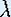Up: Introduction

# One-Dimensional Dynamical Systems

## Part 1: Introduction

#### Parameter dependency

So far, we have seen that the dynamics of our squirrel model does not depend on the choice of the initial state, at least not qualitatively, whenever x > 0. Let us now elaborate a little on the function f itself.

The function

f(x) = 3x

describes a model for which we assume that each pair of squirrels has 4 babies. What if they would have 6, or 10? What if a (constant) percentage of the population dies each year? In qualitative terms, it would not really matter: eventually we will just end up with lots of squirrels. What if each couple gets only 2 babies, and dies right away each year? Think what would happen if the number of newborns is less then the number of deaths.

We want to reflect the choice of how many babies there are born and how many squirrels die in our dynamical system. For this we use a parameter. Assuming that each year we have a constant growth rate, we can write our function as follows:

f(x) =x.

Iflies in between 0 and 1, the squirrel population will eventually die out. Ifis larger than 1, there will be infinitely many. Ifis exactly 1, the squirrel population does not change at all. We could also consider negative values for, but for the squirrel model this has no meaning.

Up: IntroductionThe Geometry Center Home Page

Written by Hinke Osinga